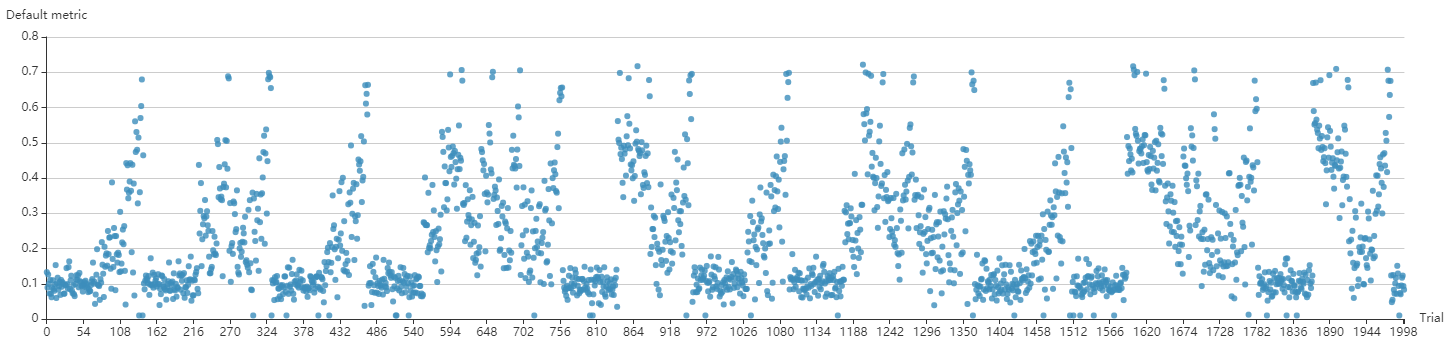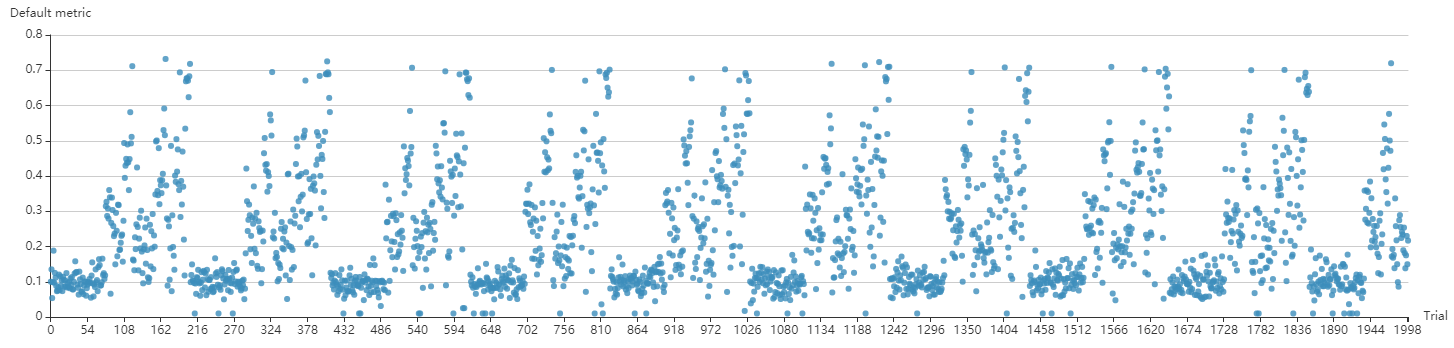Hyperband is a popular autoML algorithm. The basic idea of Hyperband is to create several buckets, each having `n` randomly generated hyperparameter configurations, each configuration using `r` resources (e.g., epoch number, batch number). After the `n` configurations are finished, it chooses the top `n/eta` configurations and runs them using increased `r*eta` resources. At last, it chooses the best configuration it has found so far.

## Implementation with full parallelism¶

First, this is an example of how to write an autoML algorithm based on MsgDispatcherBase, rather than Tuner and Assessor. Hyperband is implemented in this way because it integrates the functions of both Tuner and Assessor, thus, we call it Advisor.

Second, this implementation fully leverages Hyperband’s internal parallelism. Specifically, the next bucket is not started strictly after the current bucket. Instead, it starts when there are available resources. If you want to use full parallelism mode, set `exec_mode` with `parallelism`.

Or if you want to set `exec_mode` with `serial` according to the original algorithm. In this mode, the next bucket will start strictly after the current bucket.

`parallelism` mode may lead to multiple unfinished buckets, and there is at most one unfinished bucket under `serial` mode. The advantage of `parallelism` mode is to make full use of resources, which may reduce the experiment duration multiple times. The following two pictures are the results of quick verification using nas-bench-201, picture above is in `parallelism` mode, picture below is in `serial` mode.If you want to reproduce these results, refer to the example under `examples/trials/benchmarking/` for details.

## Usage¶

### Config file¶

To use Hyperband, you should add the following spec in your experiment’s YAML config file:

```advisor:
#choice: Hyperband
classArgs:
#R: the maximum trial budget
R: 100
#eta: proportion of discarded trials
eta: 3
#choice: maximize, minimize
optimize_mode: maximize
#choice: serial, parallelism
exec_mode: parallelism
```

Note that once you use Advisor, you are not allowed to add a Tuner and Assessor spec in the config file. If you use Hyperband, among the hyperparameters (i.e., key-value pairs) received by a trial, there will be one more key called `TRIAL_BUDGET` defined by user. By using this ``TRIAL_BUDGET``, the trial can control how long it runs.

For `report_intermediate_result(metric)` and `report_final_result(metric)` in your trial code, ``metric`` should be either a number or a dict which has a key ``default`` with a number as its value. This number is the one you want to maximize or minimize, for example, accuracy or loss.

`R` and `eta` are the parameters of Hyperband that you can change. `R` means the maximum trial budget that can be allocated to a configuration. Here, trial budget could mean the number of epochs or mini-batches. This `TRIAL_BUDGET` should be used by the trial to control how long it runs. Refer to the example under `examples/trials/mnist-advisor/` for details.

`eta` means `n/eta` configurations from `n` configurations will survive and rerun using more budgets.

Here is a concrete example of `R=81` and `eta=3`:

s=4

s=3

s=2

s=1

s=0

i

n r

n r

n r

n r

n r

0

81 1

27 3

9 9

6 27

5 81

1

27 3

9 9

3 27

2 81

2

9 9

3 27

1 81

3

3 27

1 81

4

1 81

`s` means bucket, `n` means the number of configurations that are generated, the corresponding `r` means how many budgets these configurations run. `i` means round, for example, bucket 4 has 5 rounds, bucket 3 has 4 rounds.

For information about writing trial code, please refer to the instructions under `examples/trials/mnist-hyperband/`.

### classArgs requirements¶

• optimize_mode (maximize or minimize, optional, default = maximize) - If ‘maximize’, the tuner will try to maximize metrics. If ‘minimize’, the tuner will try to minimize metrics.

• R (int, optional, default = 60) - the maximum budget given to a trial (could be the number of mini-batches or epochs). Each trial should use TRIAL_BUDGET to control how long they run.

• eta (int, optional, default = 3) - `(eta-1)/eta` is the proportion of discarded trials.

• exec_mode (serial or parallelism, optional, default = parallelism) - If ‘parallelism’, the tuner will try to use available resources to start new bucket immediately. If ‘serial’, the tuner will only start new bucket after the current bucket is done.

### Example Configuration¶

```# config.yml
The current implementation of Hyperband can be further improved by supporting a simple early stop algorithm since it’s possible that not all the configurations in the top `n/eta` perform well. Any unpromising configurations should be stopped early.